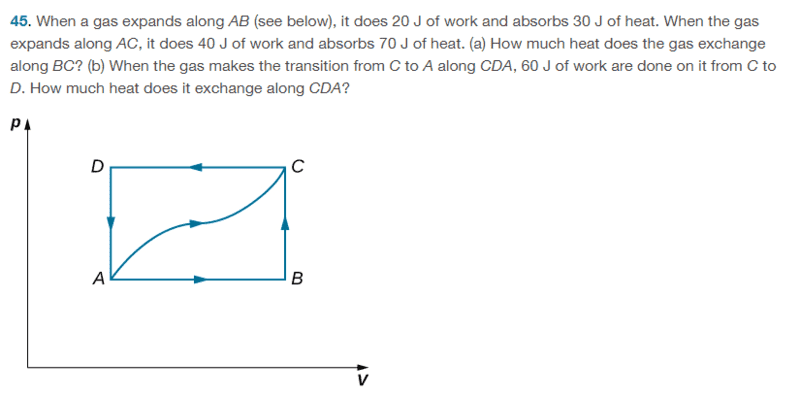# Finding heat exchanged work done to compress gas

• ChiralSuperfields

#### ChiralSuperfields

Homework Statement
For this part(b) of this problem:

When a gas expands along AB (see below), it does 20 J of work and absorbs 30 J of heat. When the gas expands along AC, it does 40 J of work and absorbs 70 J of heat. (a) How much heat does the gas exchange along BC? (b) When the gas makes the transition from C to A along CDA, 60 J of work are done on it from C to D. How much heat does it exchange along CDA?

The solution is ##90J##. However, I am getting ##30J##. My working is

##\Delta E_{int_{CDA}} = -\Delta E_{int_{CA}} = -30 ##
##-30 = Q - (-60) ##
## 30 J = Q ##

Can some please let me know what I am doing wrong?

Many thanks!
Relevant Equations
First Law of Thermodynamics ##\Delta E_{int} = Q - W##If
##-30 = Q - (-60)##,
what do you get when you move ##(-60)## to the left side and change the sign that is in front of it outside the parentheses? Alternatively, get rid of the parentheses first, then move the term over.

•MatinSAR and ChiralSuperfields
If
##-30 = Q - (-60)##,
what do you get when you move ##(-60)## to the left side and change the sign that is in front of it outside the parentheses? Alternatively, get rid of the parentheses first, then move the term over.

##-30 = Q + 60##
##-90 = Q##

But the solution is ##90 = Q##. Thank you, I guess I messed up in the algebra there, but is the reason why in their answer ##Q > 0## because there are only concerned when the heat exchanged so we don't need to say that the heat is loss from the system?

Many thanks!

•kuruman
The easiest way to do this is to take point A as the datum of zero internal energy: ##E_A=0##. Then $$E_B=30-20=10\ J$$
$$E_C=70-40=30\ J$$So $$E_A-E_C=-30=Q+60$$

•ChiralSuperfields and MatinSAR

##-30 = Q + 60##
##-90 = Q##

But the solution is ##90 = Q##. Thank you, I guess I messed up in the algebra there, but is the reason why in their answer ##Q > 0## because there are only concerned when the heat exchanged so we don't need to say that the heat is loss from the system?

Many thanks!
Yes. It's the magnitude of the heat that flows between the environment and gas regardless of where this heat starts and ends up. There are four ways to say the same thing:
1. The heat that leaves the gas and enters the environment is 90 J
2. The heat that enters the environment from the gas is 90 J.
3. The heat that leaves the environment and enters the gas is - 90 J.
4. The heat that enters the gas from the environment is -90 J.
These can be summarized with loss of direction information as "The heat that is exchanged between gas and environment is 90 J." By convention, the ##Q## in the first law of thermodynamics is chosen to represent the heat that enters the gas from the environment, option 4.

Last edited:
•ChiralSuperfields
The easiest way to do this is to take point A as the datum of zero internal energy: ##E_A=0##. Then $$E_B=30-20=10\ J$$
$$E_C=70-40=30\ J$$So $$E_A-E_C=-30=Q+60$$
Thank you for your reply @Chestermiller ! That is a good method that I have not seen before!

Yes. It's the magnitude of the heat that flows between the environment and gas regardless of where this heat starts and ends up. There are four ways to say the same thing:
1. The heat that leaves the gas and enters the environment is 90 J
2. The heat that enters the environment from the gas is 90 J.
3. The heat that leaves the environment and enters the gas is - 90 J.
4. The heat that enters the gas from the environment is -90 J.
These can be summarized with loss of direction information as "The heat that is exchanged between gas and environment is 90 J." By convention, the ##Q## in the first law of thermodynamics is chosen to represent the heat that enters the gas from the environment, option 4.